# Imaginary Numbers on the ACT

Sadly, ACT imaginary numbers aren’t the ones you may have come up with as a child, like eleventy-twelve. (Though if any of you become mathematicians one day, please make that happen. It would be fun!)

No, real-life imaginary numbers (and isn’t that a weird turn of phrase) were discovered/invented as a way to take the square root of a negative number. With real numbers, we can’t do that, but by using our imaginary number, we totally can!

Basically, if you take the square root of -1, you wind up with the imaginary number i

Translated into math, it looks like this: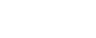Simple so far, yeah? Good, because we’re about to introduce a little complexity up in here.

## Simplifying Imaginary Numbers on ACT Math

Okay, let’s take a look at an example. Say you needed to find the square root of -16.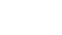Well, how do you get the number -16? One way is to multiply 16 and -1, right? So let’s rewrite it like this: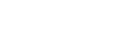We already know that √-1 is i, and you should know that √16 is 4. So now our example looks like this:Simple, right? No? Okay then, let’s try another example. What if we wanted to find √-x? Using the same steps we followed above, here’s our progression:A little more complicated now, but still totally doable. Your answer would be i√x.

## Powers of Imaginary Numbers

All right, stop looking at me like that. This is way easier than it sounds at first.

Let’s say you needed to figure out what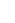is , or, to write it another way i x i. Since we already know that i is √-1, asking foris just another way of saying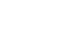. When you square a square root, both of them cancel out, leaving you with the answer of -1.

So,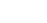.

How about? That would be . But instead of thinking of it as (√-1)*(√-1)*(√-1), think of it as building on the power we already know, namely . Sowould look like this: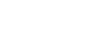which is just another way of saying

-1 x i

which is just another way of saying

-i

So,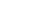.

Easy peasy so far, right? Let’s try. We can break that down like so: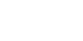As we already know,is -1. This is just a fancy way of writing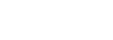which, of course, equals 1.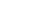Now let’s live dangerously and figure out. And remember, we’re building on what we already know, so we can rewrite it like this: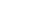We already know that, so our example now looks like this: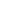Or, to simplify:

i## A Nifty Little Trick

Let’s write out everything we’ve learned so far in a list, includingTake a close look at everything we’ve found so far. The powers of i work in a very clear cycle. You can remember it with a lovely little mnemonic device that I learned in school. Say the answers we uncovered aloud, ignoring the negative signs.All you have to do is remember “I Won,” and you can figure out any power of i, any time, anywhere.

But wait! I hear you cry. What about the negative signs? Well, just remember that the negative signs are on the inside of the cycle, like so:Now that we’ve reviewed all that, let’s look at an ACT-style example.

The imaginary number i is defined such that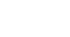. What does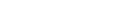equal?

1. i
2. -i
3. 0
4. -1
5. 1

I’ll give you a minute to try to puzzle this one out. If you need me, I’ll be humming the song they play on “Jeopardy!”

.

.

.

Okay, how did you do? Did you remember “I -Won, -I Won”? Let’s take a look.

The first four terms in our question work out like this:

i + -1 + -i + 1 = 0

You can count on the sum being 0 every four terms, since we’re working with a cycle. So let’s think about this logically. We’re dealing with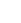at the end of our question. How many groups of four are there in 21?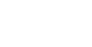As far as we’re concerned, that 0.25 left at the end is all we care about. That means we’re one term into the next cycle. And if you remember your mnemonic device, we’ve gone through “I -Won, -I Won” five times in this problem. As the first term is always i, our answer is A.

Here’s one to try on your own:

The imaginary number i is defined such that. What does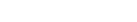equal?

1. i
2. -i
3. 0
4. i-1
5. 1-i

Remember our cycles of four. How many of those are we dealing with? 54/4 = 13.5

Disregard the first 13 cycles and just look at the 0.5 at the end. That means we’re two terms into the 14th cycle, and everything before that cancels out. i^53 = i and i^54 = -1, so the remaining sum is i-1, so our answer is D. Remember “I -Won, -I Won,” and it’s easy!

For more on imaginary numbers, check out Kristin’s explanation below!

## Author

•Catrina graduated from Rider University with a B.A. in English. She’s been helping students prepare for standardized tests since 2011. In her spare time, you can find her reading anything within arms’ reach, playing video games, correcting grammar, or studying word derivations. (Did you know that procrastinate comes from the Latin word cras, which means “tomorrow”?)